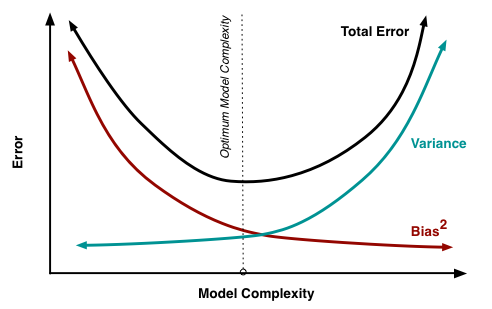# Decoding the Regularization Parameter Lambda in Machine Learning: An In-depth Exploration of its Role, Significance, and OptimizationMachine learning (ML) is an amalgamation of complex algorithms and models, fine-tuned by a set of parameters that significantly affect their performance. Among these parameters, the regularization parameter, often denoted as ‘lambda,’ plays a crucial role in controlling the balance between bias and variance in machine learning models. This comprehensive article will delve into the concept of the lambda parameter, its application in regularization, and how to effectively optimize its value for superior model performance.

### Understanding Regularization in Machine Learning

Regularization is a technique used in ML to prevent overfitting, which occurs when a model learns the training data too well, capturing the noise along with the underlying pattern. Overfit models tend to perform poorly on unseen data, as they fail to generalize well. Regularization addresses this issue by adding a penalty to the loss function, effectively limiting the complexity of the model.

There are two common types of regularization: L1 and L2 regularization, also known as Lasso and Ridge regularization respectively. L1 regularization tends to create sparser solutions, driving some feature coefficients to zero, effectively performing feature selection. L2 regularization, on the other hand, tends not to favor sparse solutions and instead leads to smaller coefficients in general.

### The Role of the Lambda Parameter

The ‘lambda’ parameter, in the context of regularization, determines the amount of shrinkage applied to a model. It controls the trade-off between bias and variance. A high lambda value increases the amount of regularization and creates a simpler model with a higher bias but lower variance. Conversely, a lower lambda decreases regularization, leading to a more complex model with lower bias but potentially higher variance.

The regularization term’s magnitude, controlled by lambda, serves to prevent the model from fitting too closely to the training data, reducing the chance of overfitting and enhancing the model’s predictive performance on unseen data.

### Strategies for Optimizing the Lambda Parameter

Determining the optimal lambda parameter is crucial to achieving the best possible model performance. The right lambda value depends on the specific dataset and model, and it is typically found through empirical methods. Here are a few strategies:

1. Cross-Validation: Cross-validation is often used to find the optimal lambda. The model is trained with different lambda values, and the model performance is evaluated using cross-validation. The lambda that results in the best cross-validation performance is typically chosen as the optimal value.

2. Grid Search: Grid search is a method of hyperparameter tuning where the model is trained with a range of lambda values. The model’s performance is evaluated for each, and the lambda that provides the best performance is chosen.

3. Regularization Path: Some ML platforms, like H2O, allow users to plot a regularization path, which shows how the model coefficients change with different lambda values. This can provide insight into the impact of lambda on the model and guide the selection of an appropriate value.

### The Lambda Parameter in Different Models

The regularization parameter lambda is an integral part of several ML models:

1. Linear Regression: In Ridge and Lasso regression, lambda controls the degree of regularization applied to the linear regression model.

2. Logistic Regression: Similar to linear regression, lambda can also be used in logistic regression for regularization.

3. Neural Networks: In neural networks, lambda is used for weight decay, a form of regularization where the weights of the network are shrunk towards zero.

4. Support Vector Machines (SVM): In SVM, a form of lambda, called ‘C’, is used as a regularization parameter. However, unlike lambda, a higher ‘C’ results in less regularization.

### Conclusion

The regularization parameter lambda is a crucial cog in the machine learning wheel, playing a vital role in improving model generalization and preventing overfitting. By understanding the concept of lambda and employing strategies such as cross-validation or grid search to optimize its value, one can build more robust, reliable, and accurate machine learning models.

As machine learning continues to revolutionize industries across the board, a deep understanding of core concepts like regularization and the lambda parameter remains essential. Through the effective use of these techniques, data scientists and ML practitioners can drive predictive accuracy, adding value to business outcomes and decision-making processes.

# Personal Career & Learning Guide for Data Analyst, Data Engineer and Data Scientist

## Applied Machine Learning & Data Science Projects and Coding Recipes for Beginners

A list of FREE programming examples together with eTutorials & eBooks @ SETScholars

# Projects and Coding Recipes, eTutorials and eBooks: The best All-in-One resources for Data Analyst, Data Scientist, Machine Learning Engineer and Software Developer

Topics included:Classification, Clustering, Regression, Forecasting, Algorithms, Data Structures, Data Analytics & Data Science, Deep Learning, Machine Learning, Programming Languages and Software Tools & Packages.
(Discount is valid for limited time only)## Find more … …

Learn Java by Example: Java Program to pass lambda expression as a method argument

Python tutorials for Business Analyst – Python Lambda Function

Machine Learning Mastery: Linear Regression Using Tensorflow